# Inductive Logic Programming - Theory## Introduction

This page provides an outline of the fundamental concepts and theory of ILP. The areas covered are:

## Inductive Inference

Inductive inference is, in a sense, the inverse of deduction. However, deductive inference proceeds by application of sound rules of inference, while inductive inference typically involves unsound conjecture.

Deductive inference derives consequences E from a prior theory T. Similarly, inductive inference derives a general belief T from specific beliefs E. In both deduction and induction T and E must be consistent and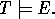Within ILP it is usual to separate the above elements into examples (E), background knowledge (B), and hypothesis (H). These have the relationship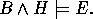B, H and E are each logic programs. E usually consists of ground unit clauses of a single target predicate. E can be separated into positive examples (E+), as ground unit definite clauses and negative examples (E-), ground unit headless Horn clauses. However, the separation into B, H and E is a matter of convenience

## Inverse Resolution

Both Logic Programming and ILP are built upon Robinson's seminal work on Resolution Theorem Proving. He demonstrated that deductive inference in the first order predicate calculus could be effected by the single Resolution rule of inference. This forms a basis for the programming system Prolog.

A single resolution step is shown below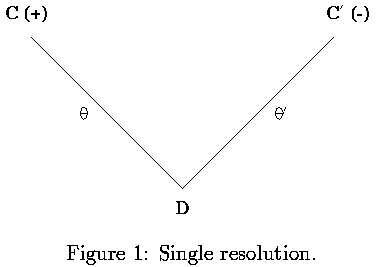Inductive inference based on inverting resolution in propositional logic was the basis of the inductive inference rules within the Duce system.

### Inductive inference rules

Duce had six inductive inference rules. Four of these were concerned with definite clause propositional logic. In the following description of the inference rules lower-case letters represent propositional variables and upper-case letters represent conjunctions of propositional variables.Duce's inference rules invert single-depth applications of resolution. Using the rules a set of resolution-based trees for deriving the examples can be constructed backwards from their roots. The set of leaves of the trees represent a theory from which the examples can be derived. In the process new proposition symbols, not found in the examples, can be ''invented'' by the intra- and inter-construction rules.

### Inverse Resolution in First Order Logic

Inverse resolution was lifted to first-order predicate calculus. This involved algebraic inversion of the equations of resolution below.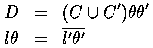Figure 1 shows a resolution step. During a deductive resolution step D is derived at the base of the 'V' given the clauses on the arms. In contrast, a 'V' inductive inference step derives one of the clauses on the arm of the `V' given the clause on the other arm and the clause at the base. In Figure 1 the literal resolved is positive (+) in C and negative (-) in C'. Duce's absorption rule constructs C' from C and D, while the identification rule derives C from C' and D.

Since algebraic inversion of resolution has a complex non-deterministic solution only a restricted form of absorption was implemented in Cigol (logiC backwards). However, there is a unique most-specific solution for `V' inductive inference rules. That iswhere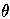is such thatRather than inverting the equations of resolution we might consider resolution from the model-theoretic point of view. That is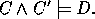Applying the deduction theorem gives a deductive solution for absorption.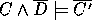This is a special case of inverting implication.

## Relative Least General Generalisation (rlgg)

One commonly advocated approach to learning from positive data is that of taking relative least general generalisations (rlggs) of clauses.

In the late 1960s Reynolds and Plotkin investigated the problem of finding least general generalisations (lggs) of atoms. The work focused on the importance of Robinson's unification to deduction, and searched for an analogue useful in induction. Lggs were found to be, in some sense, an inverse to unification.

Plotkin extended the investigation to clauses. He then went on to define the lgg of two clauses relative to clausal background knowledge B.

Assume that one is attempting to learn target concept T, from examples E={x_1,x_2,...,x_m}. Given background knowledge , H=rlgg_B (E) will be the hypothesis within the relative subsumption lattice with the fewest possible errors of commission (instances x in X for which H entails x and T does not entail x).

This approach to learning from positive data has the following problems.

• Arbitrary background knowledge. Plotkin showed that with unrestricted definite clause background knowledge B there may not be any finite rlgg_B(E).
• Extensional background knowledge. Suppose B and E consist of n and m ground unit clauses respectively. In the worst case the number of literals in rlgg_B(E) will be (n+1)^m, making the construction intractable for large m.
• Multiple clause hypothesis. Target concepts with multiple clauses cannot be learned since rlgg_B(E) is a single clause.
The ILP system Golem was designed to learn by creating rlggs. Golem used extensional background knowledge to avoid the problem of non-finite rlggs. Extensional background knowledge B can be generated from intensional background knowledge B' by generating all ground unit clauses derivable from B' in at most h resolution steps. The parameter h is provided by the user. The rlggs constructed by Golem were forced to have only a tractable number of literals by requiring that the set of possible hypotheses contain only definite clause theories that were ij-determinate.

## Inverse Implication (II)

The inability to invert implication between clauses limits the completeness of inverse resolution and rlggs since-subsumption is used in place of clause implication in both. Plotkin noted that if clause C-subsumes clause D (or) then C -> D. However, he also notes that C -> D does not imply. Although efficient methods are known for enumerating every clause C which-subsumes an arbitrary clause D, this is not the case for clauses C which imply D. This is known as the problem of inverting implication between clauses.

Gottlob proved a number of properties concerning implication between clauses. He demonstrated that for any two non-tautological clauses, C,D, where C+, C-, and D+, D- be the sets of positive and negative literals of clauses C and D respectively, then C -> D implies that C+-subsumes D+ and C--subsumes D-.

Sub-unification has been applied In an attempt to solve the inverting implication problem. Sub-unification is a process of matching sub-terms in D to produce C. It has been demonstrated that sub-unification is able to construct recursive clauses from fewer examples than would be required by ILP systems such as Golem and FOIL.

Another notable Lemma was proved by Lee. This states that a clause T implies clause C if and only if there exists a clause D in the resolution closure of T, such that-subsumes C.

In particular it is shown that Lee's subsumption lemma has the following corollary. (Implication and recursion)

Let C, D be clauses. C -> D if and only if either D is a tautology or C-subsumes D or there is a clause E such that E-subsumes D where E is constructed by repeatedly self-resolving C.

Thus the difference between-subsumption and implication between clauses C and D is only pertinent when C can self-resolve. Attempts were made to a) extend inverse resolution and b) use a mixture of inverse resolution and lgg to solve the problem. The extended inverse resolution method suffers from problems of non-determinacy. Idestam-Almquist's use of lgg suffers from the standard problem of intractably large clauses. Both approaches are incomplete for inverting implication, though Idestam-Almquist's technique is complete for a restricted form of entailment called T-implication.

It has been shown that for certain recursive clauses D, all the clauses C which imply D also-subsume a logically equivalent clause D'. Up to renaming of variables every clause D has at most one most specific form of D' in the-subsumption lattice. D' is called the self-saturation of D. However, there exist definite clauses which have no finite self-saturation.

## Inverse Entailment (IE)

The general problem specification of ILP is, given background knowledge B and examples E find the simplest consistent hypothesis H (where simplicity is measured relative to a prior distribution) such thatNote, in general B, H and E could be arbitrary logic programs. Each clause in the simplest H should explain at least one example, since otherwise there is a simpler H' which will do. Consider then the case of H and E each being single Horn clauses. This can now be seen as a generalised form of absorption and rearranged similarly to give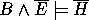Since H and E are each single clauses, their negation will be logic programs consisting only of ground skolemised unit clauses. Let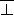be the (potentially infinite) conjunction of ground literals which are true in all models of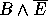. Since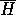must be true in every model ofit must contain a subset of the ground literals in. Thereforeand so for all HA subset of the solutions for H can be found by considering the clauses which-subsume. The complete set of candidates for H can be found by considering all clauses which-subsume sub-saturants of.

## U-Learnability

U-Learnability is a new model of learnability, and is an alternative to PAC-Learnability. U-Learnability better matches the practical goals of machine learning.

The major features of U-learnability that distinguishes it from PAC-learnability are:

1. The use of probability distributions over concept classes, which assign probabilities to potential target concepts.
2. Average-case sample complexity and time complexity requirements, rather than worst-case requirements.
In the U-learnabilty model, a teacher randomly chooses a target concept according to a probability distribution over the concept class. The teacher then chooses examples randomly, with replacement, according to a probability distribution over the domain of examples, and labels the examples according to the chosen target. In general, these distributions may be known, completely unknown, or partially known to the learner. In the case where these distributions are completely unknown, then worst case analysis must be used as in PAC-learnability.

More formally, the teacher starts by choosing distributions F and G from the family of distributions over concept descriptions H (wffs with associated bounds for time taken to test entailment) and instances X (ground wffs) respectively. The teacher uses F and G to carry out an infinite series of teaching sessions. In each session a target theory T is chosen from F. Each T is used to provide labels from (True, False) for a set of instances randomly chosen according to distribution G. The teacher labels each instance x_i in the series < x_1, .., x_m > with True if T entails x_i and False otherwise. An hypothesis H is said to explain a set of examples E whenever it both entails and is consistent with E. On the basis of the series of labelled instances < e_1, e_2, ..., e_m >, a Turing machine learner L produces a sequence of hypotheses < H_1, H_2, ... H_m > such that H_i explains { e_1, ..., e_i }. H_i must be suggested by L in expected time bounded by a fixed polynomial function of i. The teacher stops a session once the learner suggests hypothesis H_m with expected error less than e for the label of any x_m+1 chosen randomly from G. < F, G > is said to be U-learnable if and only if there exists a Turing machine learner L such that for any choice of d and e (0 < d, e =< 1) with probability at least (1-d) in any of the sessions m is less than a fixed polynomial function of 1/d and 1/e.

The figure below shows the effect E={e1,.., em} has on the probabilities associated with the possible hypotheses.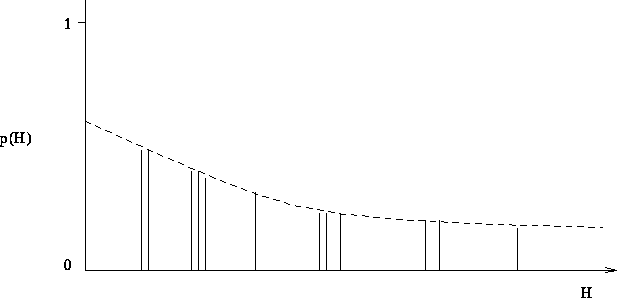U-leanability may be interpreted from a Bayesian perspective. The figure shows the effect of a series of examples on the probabilites associated with hypotheses. The learner's hypothesis language is laid out along the X-axis with prior probability p(H) = F(H) for a H taken from the set of all hypotheses measured along the Y-axis, where the sum of all probabilites of the hypotheses is 1.

The descending dotted line in the Figure represents a bound on the prior probabilities of hypotheses before consideration of examples E. The hypotheses which entail and are consistent with the examples are marked as vertical bars. The prior probability of E, p(E), is simply the sum of probabilities of hypotheses in that explain the examples. The conditional probability p(E|H) is 1 in the case that H explains E and 0 otherwise. The posterior probability of H is now given by Bayes theorem as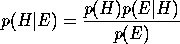For an hypotheses H which explains all the data, p(H|E) will increase monotonically with increasing E.

U-learnability has demonstrated positive results for the popular decision tree learning program, CART.

## Current Research Issues

This section provides a breif outline of the reseach areas that will extend current ILP theory and systems.

### Background Knowledge.

• Relevance. When large numbers of background predicates are available, determining which predicate is relevant.
• Revision. How clauses should be removed, or unfolded with respect to deep-structured theories.
• Invention. Further research is required into predicate invention.

### Complex Theories.

• Multi-clause. Most present ILP systems are concerned with generating a single clause. Research is required into improved performance of multiple clause generation.
• Deep Theories. Current ILP systems perform poorly in the presence of relevant long chains of literals, connected by shared variables.
• Recursion. Recursive hypotheses are poorly handled by most ILP system. This is particularly important in the natural language domain.
• Structure. Structural concepts result in complex clauses when encoded as literals. These present problems to the search strategies used in current ILP systems.

### Built-in semantics.

• Numbers. ILP systems have severe restrictions on the form of numeric constraints that can be used.
• Probabilities. ILP systems lack the ability te express probabilistic constraints. This effects the performance of ILP systems in the database discovery domain.
• Constraints. ILP system require the ability to learn and make use of general constraints, rather than requiring large numbers of ground negative examples.
• Built-in predicates. Some predicates are best defined procedurally. ILP systems may experience improved efficiency of learning using built-in predicates.

### Sampling Issues.

• Large Data Sets. Incremental learning systems may be more effective than batch systems, where difficulties are experienced with learning from all examples at once.
• Small Data Sets. Statistical tests for significance break down when learning from small data sets. ILP systems need to demonstrate high predictive accuracy with samll training sets.
• Reliability. Extending ILP systems to indicate reliability estimates when exact generalisations are not possible.Home Page